# Peripateticism

Yuens' blog

View the Project on GitHub

# 高级凸优化

## 1. 凸函数性质

$f''(x) \geq 0$

• 一阶导大于0，则递增；一阶导小于0，则递减，一阶导等于0，则不增不减；
• 一阶倒数是自变量的变化率，二阶倒数是一阶导数的变化率。也就是一阶导数变化率的变化率；
• 连续函数的一阶导数就是相应的切线斜率；
• 二阶导数可以反应图像的凹凸。二阶导数大于0，图像为凹，二阶导数小于0，图像为凸，二阶导数等于0，不凸不凹。
• 结合一阶、二阶导数可以求函数的极值。当一阶导数等于0，而二阶导数大于0时，为极小值；当一阶导数等于0，而二阶导数小于0时，为极大值点，当一阶导数、二阶导数都等于0时，为驻点。

1. 亚线性收敛（Sub-linear rate of convergence） $$f(x^{(t)}) f(x^*) \leq O(\frac{1}{\sqrt(t)})$$

2. 线性收敛（linear rate of convergence）：如果被这个bound住，叫做线性收敛。

$f(x^{(t)}) f(x^*) \leq O(\alpha^{t}), \alpha \in (0,1)$

$f(x^{(t)}) f(x^*) \leq O(\frac{1}{\sqrt(t)}) \leq O(\alpha^{t}), \alpha \in (0,1)$

1. 随着$t$增加，右边越来越小，会越来越逼近$x$的最优值$f(x^*)$（最优解）。
2. 两个收敛率是有优劣之分，sub-linear比linear收敛要更慢一些（下面是两个函数的plot的结果）。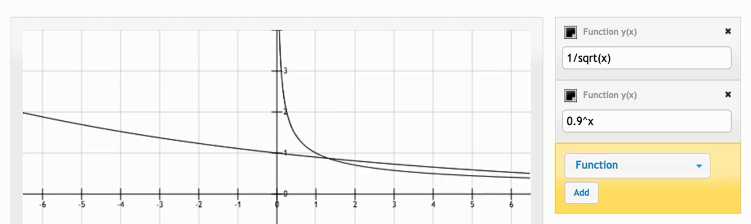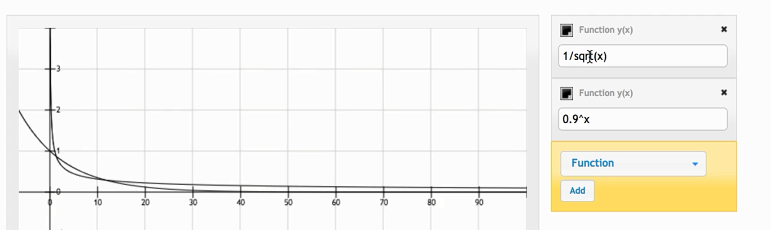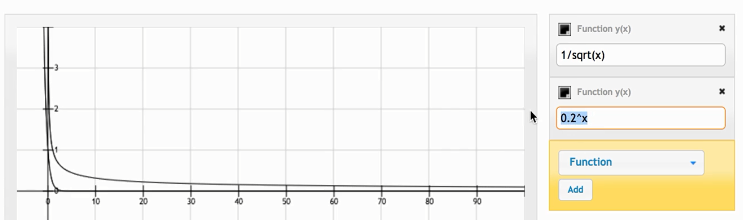## 2. 梯度下降

$E\bigtriangledown f_i(x) = \frac{1}{n}\sum_{i=1}^{n}\bigtriangledown f_i(x)$

$E \eta \bigtriangledown f_i(x) = \frac{1}{n}\sum_{i=1}^{n}\bigtriangledown f_i(x)$

### 2.1 weight decay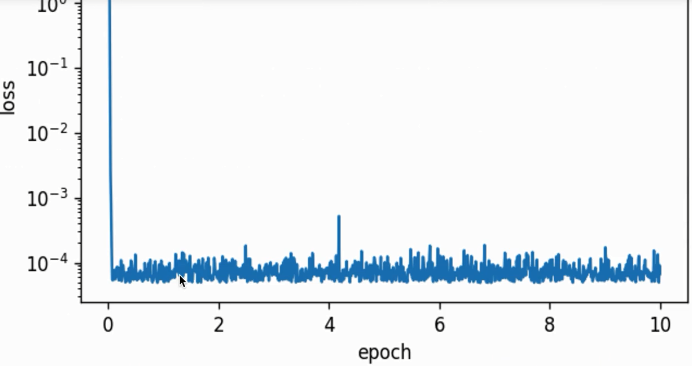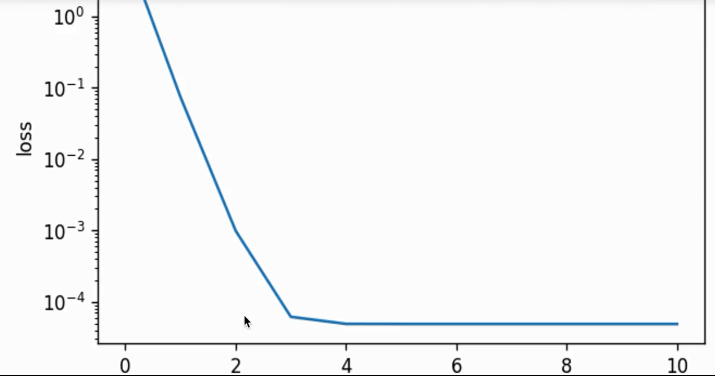lr decay=0.1，batch size=1，第二个epoch开始衰减，整个过程一开始variance较大但后面稳定，且参数的结果收敛了没再变化：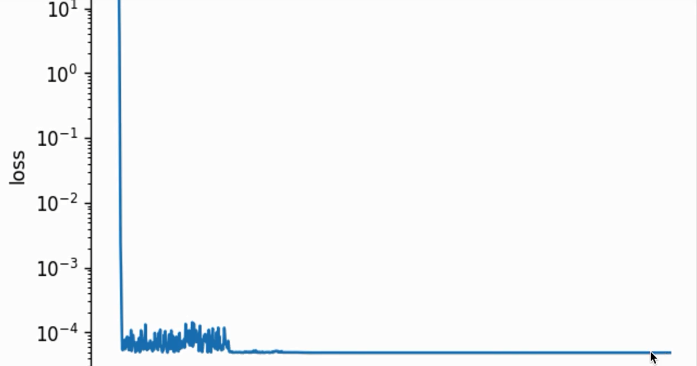## 3. 理解梯度

$\begin{bmatrix} \frac{\partial f}{\partial x_1} \\ \frac{\partial f}{\partial x_2} \end{bmatrix} = \begin{bmatrix} 2x_1x_2 \\ x_1^2 + 3e^{x_2} \end{bmatrix} = \begin{bmatrix} 4 \\ 1+3e^2 \end{bmatrix}$

## 4. 梯度方向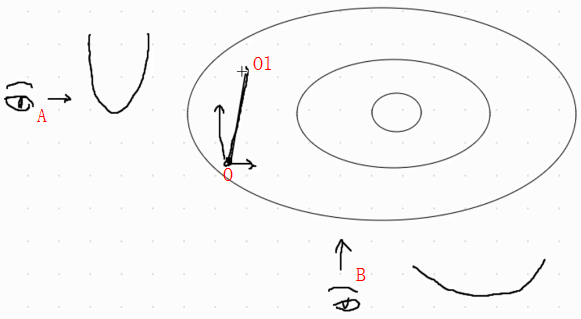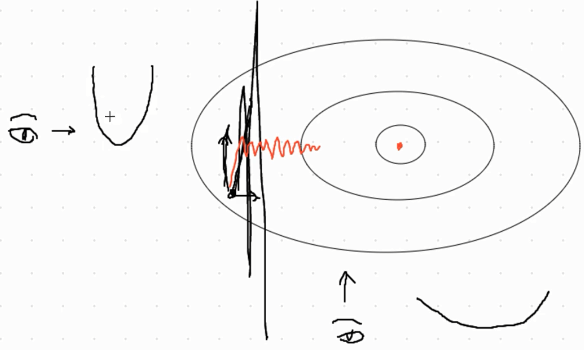## 5. 动量法（momentum）

$\overrightarrow{v} := \gamma \overrightarrow{v} + \eta \bigtriangledown f_\beta (\overrightarrow{x})$

$\overrightarrow{x} := \overrightarrow{x} - \overrightarrow{v}$

$x$是模型参数，如果上面第一个公式中$\gamma=0$的话，那么得到的$\overrightarrow{x} := \overrightarrow{x} - \overrightarrow{v} = \overrightarrow{x} - \eta \bigtriangledown f_\beta (\overrightarrow{x})$就是一个随机梯度下降。那么这个$\gamma$有啥意义呢？

$\overrightarrow{v} = \gamma \overrightarrow{v} + (1-\gamma) \frac{\eta}{(1-\gamma) } \bigtriangledown f_\beta (\overrightarrow{x})$

### 5.1 指数平均数指标

$y^{(t)} = \gamma y^{(t-1)} + (1-\gamma) x^{(t)}$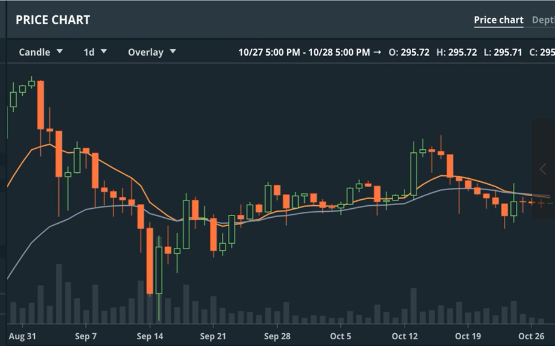$y^{(t)} = \gamma y^{(t-1)} + (1-\gamma) x^{(t)}$

\begin{align}y^{(20)} & = 0.05 x ^{20} + 0.95y^{(19)} \\ & =0.05 x^{20} + 0.05 \cdot 0.95 x^{(19)} + 0.95^{2} y^{(18)} \\ & = \dots \dots \dots \dots \dots \dots \dots \dots \dots \dots \dots \dots + 0.05 \cdot 0.95^2 x^{(18)} + 0.05 \cdot 0.95^3 \cdot x^{(17)} + \dots \end{align}

$\varepsilon^{\frac{1}{1-\varepsilon }} \approx \frac{1}{e}$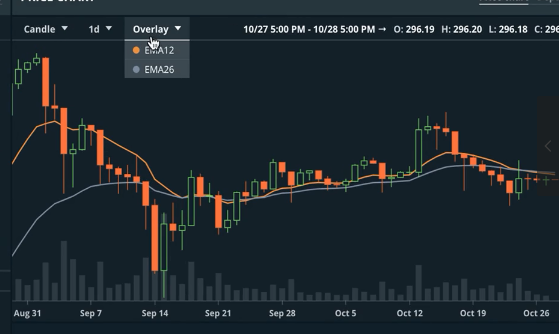EMA12和EMA26也就是对过去12天和26天做平滑平均（指数加权平均）。在后面这个EMA的格式我们会反复讲到。其实动量也可以理解为：对$\frac{\eta}{1-\gamma} \bigtriangledown f_{\beta} (\overrightarrow{x})$来做EMA，因为我们把动量$\overrightarrow{v}$写成了EMA的形式：

$\begin{eqnarray} \overrightarrow{v} & = \gamma \overrightarrow{v} + \eta \bigtriangledown f_\beta (\overrightarrow{x}) \\ & = \gamma \overrightarrow{v} + (1-\gamma) \frac{\eta}{(1-\gamma) } \bigtriangledown f_\beta (\overrightarrow{x}) \end{eqnarray}$

### 5.2 动量法的感性认识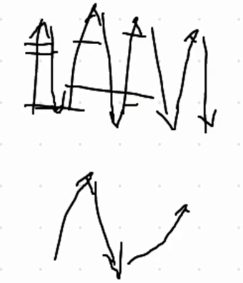### 5.3 另一种理解方式

\begin{eqnarray} \overrightarrow{v} & = \gamma \overrightarrow{v} + \eta \bigtriangledown f_\beta (\overrightarrow{x}) \\ \overrightarrow{x} & = \overrightarrow{x} - \overrightarrow{v} \end{align}

$\begin{eqnarray} \overrightarrow{v_1} &= \eta \overrightarrow{g} \\ \overrightarrow{v_2} &= \eta \overrightarrow{v_1} + \eta \overrightarrow{g} = \eta \overrightarrow{g} (\eta + 1) \\ \overrightarrow{v_3} &= \dots \dots \dots = \eta \overrightarrow{g} (\eta^2 + \eta + 1) \\ \overrightarrow{v_{inf}} &= \frac{\eta \overrightarrow{g}}{1-\gamma} \end{eqnarray}$

### 5.4 代码实现

\begin{eqnarray} \overrightarrow{v} &= \gamma \overrightarrow{v} + \eta \bigtriangledown f_\beta (\overrightarrow{x}) \\ \overrightarrow{x} &= \overrightarrow{x} - \overrightarrow{v} \end{align}

def sgd_momentum(param, vs, lr, mom, batch_size):
for param, v in zip(params, vs):
v[:] = mom * v + lr * param.grad / batch_size
param[:] -= v


### 5.5 调参说明

1. $\gamma=0.9$时，可以看到（下图）整体走势还是比较猛的，相当于当前的梯度被放大了10倍。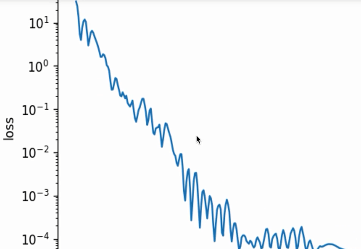2. $\gamma=0.99$时，会放大100倍，会走的更猛（下图）。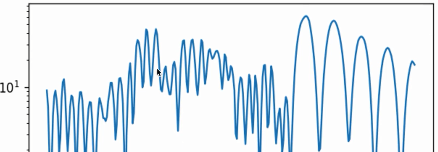3. $\gamma=0.5$时，会放大2倍，此时就很缓和了（下图）。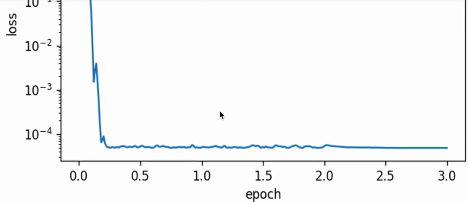$\begin{eqnarray} x_1 &:= x_1 - \eta \frac{\partial L}{\partial x_1} \\ x_2 &:= x_2 - \eta \frac{\partial L}{\partial x_2} \end{eqnarray}$

Adagrad就是一个在迭代中不断自我调整学习率，并让模型参数中每个元素使用不同学习率的。不过在介绍该算法前，需要先介绍一些按元素操作的例子，以$x = [4,9]^T$为例：

• 按元素相加：$x +1 = [5, 10]^T$
• 按元素相乘：$x \odot x = [16, 81]^T$
• 按元素相除：$72/x = [18, 8]^T$
• 按元素开方：$\sqrt{x} = [2, 3]^T$

$\begin{eqnarray} \overrightarrow{s} & = \overrightarrow{s} + \overrightarrow{g} \odot \overrightarrow{g} \\ \overrightarrow{g}^{'} & = \frac{\eta}{\sqrt{\overrightarrow{s} + \epsilon} } \odot \overrightarrow{g} \\ \overrightarrow{x} & = \overrightarrow{x} - \overrightarrow{g}^{'} \\ \end{eqnarray}$
• 公式1：$\overrightarrow{s}$是一个不断累加的公式，$\overrightarrow{g}$是模型参数为$x$时的梯度$\bigtriangledown f_{\beta} (\overrightarrow{x})$，同时$\odot$是按照元素相乘的操作；
• 公式2：真正使用的梯度是需要做一个修改的$\overrightarrow{g}^{‘}$（注意是带了一撇的$\overrightarrow{g}$），同时$\overrightarrow{s}$会被丢到开方里，再加入一个$\epsilon$来保证数值稳定性（一般我们会把$\epsilon$设置为一个比较小的常数，如adagrad常用的$\epsilon = 10^{-7}$），再按照元素相乘$\overrightarrow{g}$。其中$\eta$是初始学习率。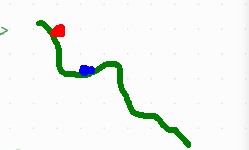adagrad因为$\overrightarrow{s}$会不断累加$\overrightarrow{s} = \overrightarrow{s} + \overrightarrow{g} \odot \overrightarrow{g}$，会导致学习率会不断衰减$\overrightarrow{g}^{‘} = \frac{\eta}{\sqrt{\overrightarrow{s} + \epsilon} } \odot \overrightarrow{g}$。说白了就是有时运气好，初始化位置好可以拿到一个不错的performance，但有时会运气不好（初始化位置不好），就糟糕了。

### 6.1 代码实现

$\begin{eqnarray} \overrightarrow{s} & = \overrightarrow{s} + \overrightarrow{g} \odot \overrightarrow{g} \\ \overrightarrow{g}^{'} & = \frac{\eta}{\sqrt{\overrightarrow{s} + \epsilon} } \odot \overrightarrow{g} \\ \overrightarrow{x} & = \overrightarrow{x} - \overrightarrow{g}^{'} \\ \end{eqnarray}$

def adagrad(params, sqrs, lr, batch_size):
eps_stable = 1e-7
for param, sqr in zip(params, sqrs):
sqr[:] += nd.squre(g)
div = lr * g / nd.sqrt(sqr + eps_stable)
param[:] -= div


## 7. RMSProp

### 7.1 代码实现

def rmsprop(params, sqrs, lr, gamma, batch_size):
eps_stable = 1e-8
for param, sqr in zip(params, sqrs):
sqr[:] = gamma * sqr + (1. - gamma) * nd.square(g)
div = lr * g / nd.sqrt(sqr + eps_stable)
param[:] -= div


### 7.2 调参说明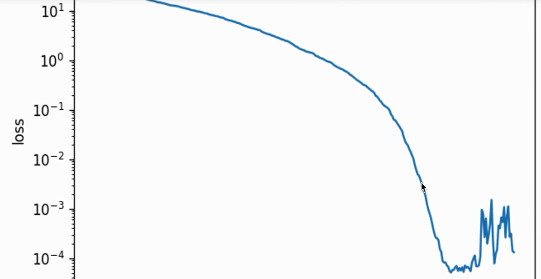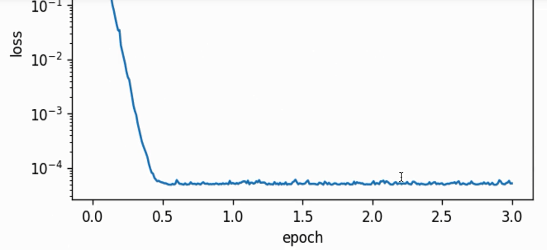$\begin{eqnarray} \overrightarrow{s} & := \gamma \overrightarrow{s} + (1 - \gamma) \overrightarrow{g} \odot \overrightarrow{g} \\ \overrightarrow{g}^{'} & := \frac{\sqrt{ \Delta \overrightarrow{x} + \epsilon }}{\sqrt{\overrightarrow{s} + \epsilon} } \odot \overrightarrow{g} \end{eqnarray}$

$\Delta x := \rho \Delta x + (1 - \rho) \overrightarrow{g}^{'} \odot \overrightarrow{g}^{'}$

### 8.1 代码实现

def adadelta(params, sqrs, delta, rho, batch_size):
eps_stable = 1e-5
for param, sqr, delta in zip(params, sqrs, deltas):
sqr[:] = rho * sqr + (1. - rho) * nd.square(g)
cur_delta = nd.sqrt(delta + eps_stable) / nd.sqrt(sqr + eps_stable)
delta[:] = rho * delta + (1. - rho) * cur_delta * cur_delta
param[:] -= cur_delta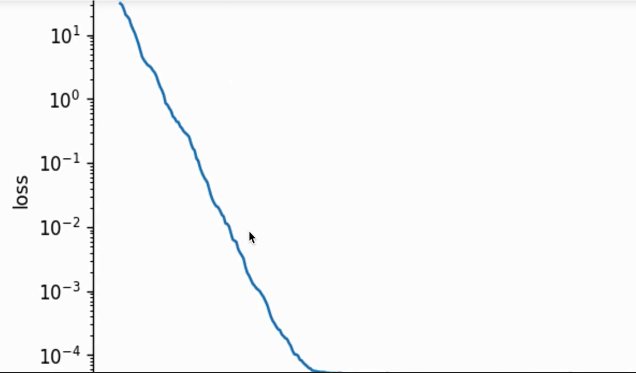Adam算法会使用一个动量变量$\overrightarrow{v}$和一个RMSProp中梯度按照元素平方的指数加权移动平均变量$\overrightarrow{s}$，并将它们中的每个元素都初始化为$0$。在每次迭代中，首先计算小批量梯度$\overrightarrow{g}$，并递增迭代次数：

$$t:=t+1$$ 然后对梯度做指数加权移动平均并计算动量变量$\overrightarrow{v}$（这里其实抄袭了一个动量法）： $$\overrightarrow{v} := \beta_1 \overrightarrow{v} + (1 - \beta_1) \overrightarrow{g}$$

$\overrightarrow{s} := \beta_2 \overrightarrow{s} + (1 - \beta_2) \overrightarrow{g} \odot \overrightarrow{g}$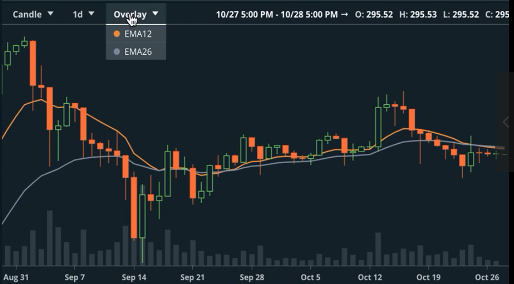$\begin{eqnarray} \hat{\overrightarrow{v}} & := \frac{\overrightarrow{v}}{1 - \beta_{1}^t} \\ \hat{\overrightarrow{s}} & := \frac{\overrightarrow{s}}{1 - \beta_{2}^t} \end{eqnarray}$

$\overrightarrow{g}^{'} := \frac{\eta \hat{\overrightarrow{v}}}{\sqrt{\hat{\overrightarrow{s}} + \epsilon}}$

$\overrightarrow{x} := \overrightarrow{x} - \overrightarrow{g}{'}$

$\begin{eqnarray} t & :=t+1 \\ \overrightarrow{v} & := \beta_1 \overrightarrow{v} + (1 - \beta_1) \overrightarrow{g} \\ \overrightarrow{s} & := \beta_2 \overrightarrow{s} + (1 - \beta_2) \overrightarrow{g} \odot \overrightarrow{g} \\ \hat{\overrightarrow{v}} & := \frac{\overrightarrow{v}}{1 - \beta_{1}^t} \\ \hat{\overrightarrow{s}} & := \frac{\overrightarrow{s}}{1 - \beta_{2}^t} \\ \overrightarrow{g}^{'} & := \frac{\eta \hat{\overrightarrow{v}}}{\sqrt{\hat{\overrightarrow{s}} + \epsilon}} \end{eqnarray}$

def adam(params, vs, sqrs, lr, batch_size, t):
beta1 = 0.9
beta2 = 0.999
eps_stable = 1e-8
for param, v, sqr in zip(params, vs, sqrs):
v[:] = betal * v + (1. - beta1) * g
sqr[:] = beta2 * sqr + (1. - beta2) * nd.square(g)
v_bias_corr = v / (1. - beta1 ** t)
sqr_bias_corr = sqr / (1. - beta ** t)
div = lr * v_bias_corr / (nd.sqrt(sqr_bias_corr) + eps_stable)
param[:] = param - div


## 10. 总结赠诗一首

• 第二句：小批量梯度下降引入动量（指数加权平均，EMA）后带来了一定抵消，Adagrad的学习率相比梯度下降（SGD，GD，mini-batch GD）有自适应模型参数元素（通过$\overrightarrow{g} \odot \overrightarrow{g}$实现）；
• 第三句：（因为Adagrad还有SGD因为lr不断decay的问题引入两个算法，其中之一的）Adadelta没有学习率（不需要手动设置初始学习率），RMSProp（是另一个解决学习率不断衰减的问题，在Adagrad基础上略作修改，把原本有关$\overrightarrow{s}$的不断累加的形式修改为EMA的形式，同时过$\overrightarrow{g} \odot \overrightarrow{g}$保留模型参数中各自元素自适应学习率的特点）；
• 第四句：Adam最后结合动量法和RMSProp（优势明显，动量法来方差的减小抵消，RMSProp带来模型参数不同元素的不同学习率和将累加的$\overrightarrow{s}$变为EMA形式不会使得学习率不断衰减）。